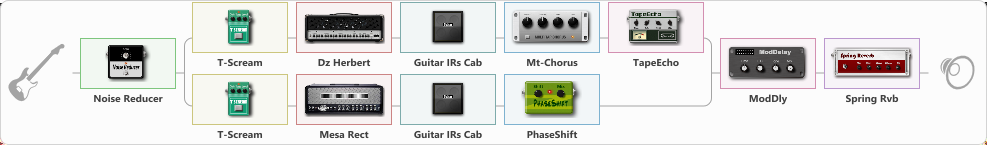# The cult tone

Discussion in 'ToneLib-GFX presets' started by truss van halen, Dec 9, 2020.

1. ### truss van halenWell-Known Member

The cult tone

Preset name: The cult tone

Effects chain:Effect: "Noise Reducer" (Dynamics / Filter), active - "yes"
{
"Sens" = 91
"Mode" = Hard
}

Effect: "Splitter" (Dynamics / Filter), active - "yes"
{
"A-Bypass" = Off
"A-Pan" = 65
"A-Level" = 66
"B-Bypass" = Off
"B-Pan" = -65
"B-Level" = 66

'A' branch:
{

Effect: "T-Scream" (Overdrive / Distortion), active - "yes"
{
"Drive" = 0
"Tone" = 71
"Level" = 82
}

Effect: "Dz Herbert" (Amp simulators), active - "yes"
{
"Gain" = 50
"Bass" = 50
"Middle" = 50
"Treble" = 50
"Presence" = 50
"Master" = 50
"Level (dB)" = 0
}

Effect: "Guitar IRs Cab" (Cabinets), active - "yes"
{
"Model" = Mesa Boogie Mk3 (1x12")
"Mic Position" = Middle
"Mic Distance" = Far
"Low Cut (Hz)" = 0
"Hi Cut (kHz)" = 20.0
"Mix" = 100
"Level (dB)" = 0
}

Effect: "Mt-Chorus" (Modulation / Sfx), active - "yes"
{
"Speed" = 1.8
"Depth" = 81
"Time" = 1.4
"Mix" = 56
}

Effect: "TapeEcho" (Delay), active - "yes"
{
"Time" = 577
"Feedback" = 7
"Tone" = 65
"LoDamp" = 19
"Mix" = 46
}
}
'B' branch:
{

Effect: "T-Scream" (Overdrive / Distortion), active - "yes"
{
"Drive" = 0
"Tone" = 71
"Level" = 82
}

Effect: "Mesa Rect" (Amp simulators), active - "yes"
{
"Gain" = 50
"Bass" = 50
"Middle" = 50
"Treble" = 50
"Presence" = 50
"Master" = 50
"Level (dB)" = 0
}

Effect: "Guitar IRs Cab" (Cabinets), active - "yes"
{
"Model" = Mesa Rectifier (4x12")
"Mic Position" = Center
"Mic Distance" = Middle
"Low Cut (Hz)" = 0
"Hi Cut (kHz)" = 20.0
"Mix" = 100
"Level (dB)" = 0
}

Effect: "PhaseShift" (Modulation / Sfx), active - "yes"
{
"Shift" = 55
"Mix" = 100
}
}
}

Effect: "ModDly" (Delay), active - "yes"
{
"Time" = 804
"Feedback" = 10
"Tone" = 66
"Speed" = 1.7
"Mix" = 38
}

Effect: "Spring Rvb" (Reverberation), active - "yes"
{
"Time" = 7.0
"PreDelay" = 0
"LoDamp" = 16
"HiDamp" = 26
"Mix" = 42
}

Note: You will need to download and install the ToneLib-GFX software to use the preset.

#### Attached Files:

• ###### The_cult_tone.tlgfx
File size:
1.2 KB
Views:
2,880
Warnak, Elijah Williams, ARSZ and 7 others like this.